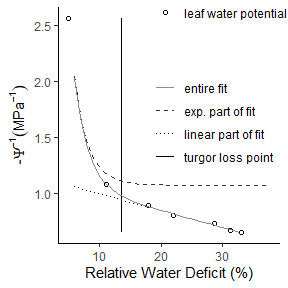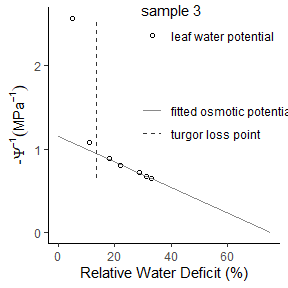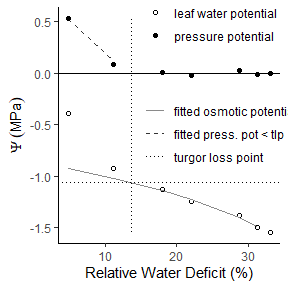A toolbox for the analysis of pressure volume curves

Experimentally obtained pressure volume curves are widely used for understanding and predicting the strategies of plants to cope with drought stress. By the use of this package, the efficient and reproducible analysis and display of entire experimental datasets is enabled. The methodology of analysing pressure volume curves is well worked out (e.g. MPatlett et al. 2012) and has been adapted by this package.

The package contains a dataset obtained experimentally on Brassica oleracea var. gongylodes for the manufacturing of pressure volume curves:

pressure_volume_data
weather_data

Pressure volume curve analysis routine

Pressure volume curves are determined by plotting inversely transformed water potential $$(-1/MPa)$$ as a function of relative water deficit ($$RWD$$, $$percentage$$). To determine the turgor loss point by the use of this package, calculate $$RWD$$ first:

pv <- RelativeWaterDeficit(pressure_volume_data)

$$RWD$$ is calculated as:

$$RWD = 100 - 100 * ((FM - DM) (FMs - DM)^{-1})$$

whereas $$FM$$ = fresh mass, $$DM$$ = dry mass and $$FMs$$ = fresh mass at water saturation.

Before continuing, check the raw data for an initial plateau. Data points in the initial part of the water potential versus RWD plot with a stronger then expected decline need to be omitted. Otherwise, the results are biased and fitting might not succeed.

Saturated Fresh Mass

In cases where the highest measured fresh mass does not equal the turgid mass, calculation of saturated fresh mass by the function FMSaturated is recommended. The function fits fresh mass values above an approximated turgor loss point linearly to water potential. The point where water potential of the linear regression line is zero equals the saturated water content.

df <- pressure_volume_data
FMSaturated(df)

Turgor Loss Point

The function TurgorLossPoint fits the data using the Gauss-Newton algorithm of nls() to a combined exponential and linear model. The exponential and linear parts are extracted and RWD at turgor loss point is localized at their point of minimum distance. The result is outputted as a list and a plot:

pv <- RelativeWaterDeficit(pressure_volume_data)
TurgorLossPoint(pv)Osmotic Potential

The function OsmoticPot allows the calculation of water potential at turgor loss point, osmotic potential at full saturation and apoplastic fraction.

First, RWD at turgor loss point is derived by the function TurgorLossPoint. The osmotic potential is then derived by fitting a linear regression line with the Gauss-Newton algorithm of nls() to the water potential data following the turgor loss point. The y- and x-axis intercept of the regression line gives the osmotic potential at full hydration (op.full.sat) and the RWD at zero 1/-Psi, respectively. RWD at zero 1/-Psi is then transferred to RWC at zero 1/-Psi to derive apoplastic fraction #’ (apo.fract) . Water potential at turgor loss point equals the value of the osmotic potential fit at the relative water deficit at turgor loss point:

pv <- RelativeWaterDeficit(pressure_volume_data)
OsmoticPot(pv)Modulus of Elasticity

Modulus of elasticity can be determined by the function ModElasticity. Relative water deficit at turgor loss point and osmotic potential are first calculated via the functions ‘TurgorLossPoint’ and ‘OsmoticPot’.

Pressure potential is derived by subtracting osmotic potential from water potential. The part of the pressure potential prior the turgor loss point is then fitted linearly and after transforming RWD (%) to RWC the modulus of elasticity (M.Elasticity) equals the slope of the fitted line:

ModElasticity(pv)